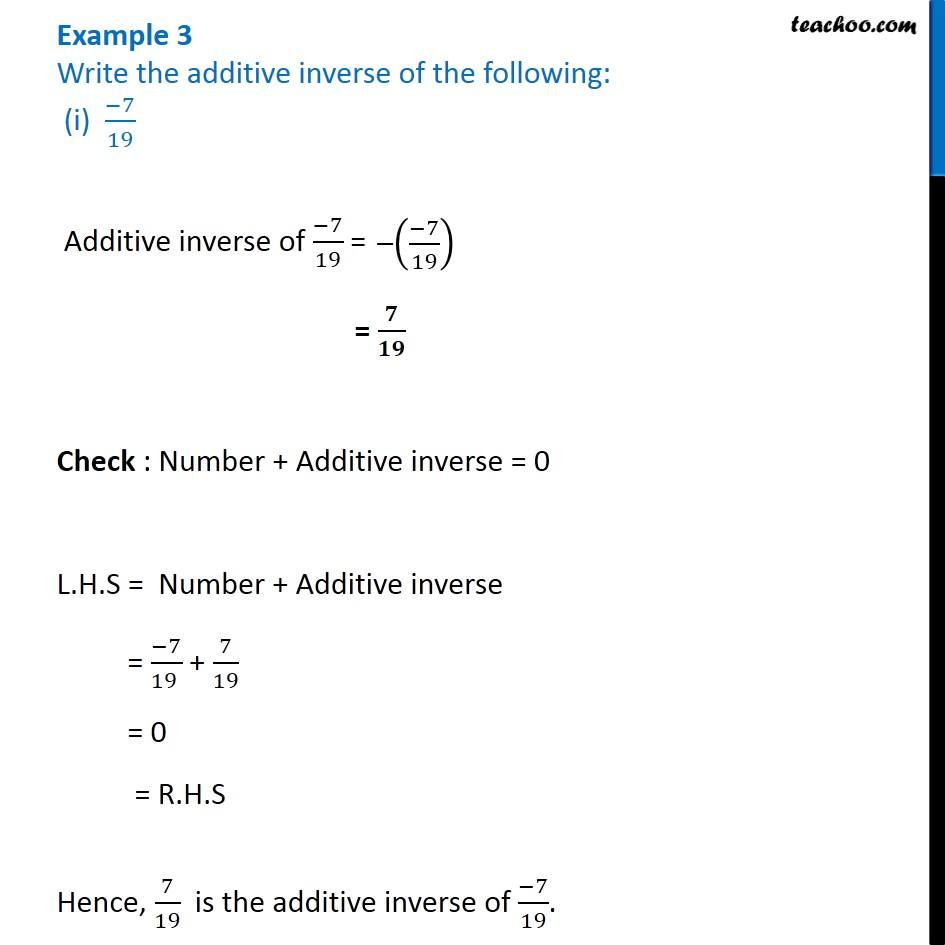Chapter 1 Class 8 Rational Numbers
Concept wiseLearn in your speed, with individual attention - Teachoo Maths 1-on-1 Class

### Transcript

Example 3 Write the additive inverse of the following: (i) (−7)/19 Additive inverse of (−7)/19 = = 𝟕/𝟏𝟗 Check : Number + Additive inverse = 0 L.H.S = Number + Additive inverse = (−7)/(19 ) + 7/19 = 0 = R.H.S Hence, 7/19 is the additive inverse of (−7)/19.